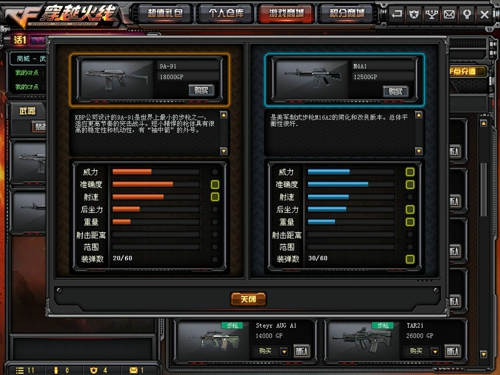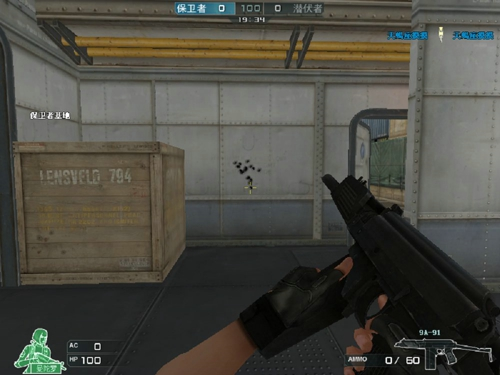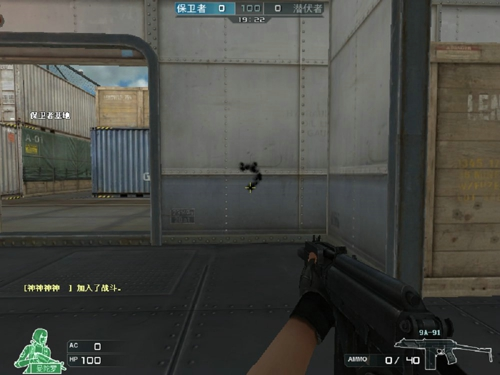# GP神器9A91武器测评

?? ? 今天小编带给大家GP神器9A91的武器测评。不过作为GP神器之一，9A91也是少数有军衔限制的武器之一。

\r

????在游戏商城中它的介绍是：KBP游戏公司设计的9A91，是世界上最小的步枪之一，适用于高节奏的突击战斗，短小精悍的枪体具有很高的稳定性和机动性，有“袖中箭”的外号。装弹数20/60，售价18000GP。通过以上介绍，我相信大家对该武器基本信息有一定的了解了吧！但它具体有哪些详细属性呢？我们还不了解，接下来小编带你具体了解一下吧！

\r

\r

1.武器伤害测评

\r

\r

\r

\r

\r

\r

\r

\r

\r

\r

\r

\r

\r

\r

\r

\r

\r

\r

\r

\r

\r

\r

\r

\r

 \r ? \r \r 有AC \r \r 无AC \r \r 头部 \r \r 89 \r \r 100 \r \r 胸部 \r \r 18 \r \r 25 \r \r 脚部 \r \r 18 \r \r 18 \r

\r

?

\r

?\r

????从数据中，我们可以看出，9A91相比其他武器伤害较低一点，毕竟属于突击步枪，威力低，但它还有其他武器没有的优势，接下来小编详细说一下。

\r

2.武器速度测评

\r

????大家都知道9A91有着极快的切换速度和换弹速度，但是相信大家没有亲身实验测试过，它究竟快到什么地步呢？今天我就使用秒表来进行时间测试，最后得出以下的速度数据，并且与之前我找到的M4A1及AK47相对比：

\r

\r

\r

\r

\r

\r

\r

\r

\r

\r

\r

\r

\r

\r

\r

\r

\r

\r

\r

\r

\r

\r

\r

\r

\r

\r

\r

\r

 \r ? \r \r 切换速度 \r \r 换弹速度 \r \r 射速 \r \r 9A91 \r \r 1.0s \r \r 1.41s \r \r 12发/秒 \r \r M4A1 \r \r 1.11s \r \r 2.20s \r \r 11发/秒 \r \r AK-47? \r \r 1.10s \r \r 2.41s \r \r 10发/秒 \r

\r

?

\r

3.弹道分析

\r

3.1站立时的弹道，如图：

\r

?\r

3.2下蹲时的弹道，如图：

\r\r

\r

\r

\r

\r

??由于子弹少，你不能随便开枪，没等打几发，子弹就没了（小编个人建议最好买个弹夹），所以，必须用最少的子弹杀掉敌人。

\r

???充分利用好你的耳麦，听你附近人的位置，离你有多远，提前预判一下敌人的位置，在什么位置应该先杀哪个后杀哪个。

\r

????在远距离对枪时，不能激动，稳扎稳打，不要一个劲的突突，要学会点射，还要不断地移动，不要在一条直线上移动，成S型移动，同时还要充分利用好你旁边掩体，来减少血量的损失。

\r

????以上就是小编对GP神器-9A91的了解，希望我的心得能给大家带来帮助.

TanSEO博客 » GP神器9A91武器测评